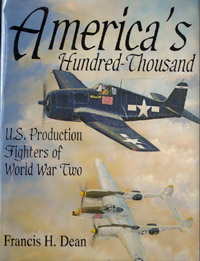## Reference Details for America's Hundred ThousandTitle America's Hundred Thousand Authors Dean Publisher Schiffer Year 1997 Types Covered Curtiss Orenco D, Vought VE-7, Boeing MB-3A, SE5A (SE5E), Curtiss TS-1, Boeing XPW-1 (PW-1), PW-8, PW-9, FB, P-1, F6C, F2B, F3B, F4B, P-12, Boeing 218, F8C Helldiver, P-16, P-6, FF, F11C, Hawk II, BFC, BF2C, F2C, Hawk III, P-26, F2F, P-30, PB-2A, F3F, Gulfhawk II (Grumman G-22), P-35, Seversky S-2 racer, Seversky EP-1, Hawk 75 (Curtiss 75, H75), P-36, Seversky AP-4, P-43, CW-21, P-66 (Vultee 48 Vanguard), P-24, P-25, P-31, XF7B (F7B), P-29, Northrop XFT (FT), Vought V-141, XP-37 (YP-37, P-37), Seversky XNF-1 (NF), XP-41 (P-41), Seversky AP-9, XP-42 (P-42), XF5F (F5F), Bell XFL-1 (FL), XP-46 (P-46), XP-50 (P-50), XP-60 (P-60), XP-49 (P-49), XP-54 (P-54), XP-56 (P-56), XP-55 (P-55), XP-75 (P-75), XP-62 (P-62), XP-67 (P-67), XP-72 (P-72), XP-77 (P-77), XF14C (F14C), XP-58 (P-58), XF8B (F8B), XF15C (F15C), P-38, P-39 (P-400), P-40, P-47, P-51 (A-36), P-61, P-63, F2A, F4F (FM), F4U, F6F Cockpit Details Brewster 339D [export F2A] (p. 452), F2A-3 (p. 464), F4F-4 (p. 501, 502, 506), FM-2 (p. 502, 503, 506), F4U-1A/D (p. 546, 547), F4U-4 (p. 548, 549), F6F (p. 579, 590), F6F-3 (p. 585, 586), F6F-5 (p. 586, 587), P-38E (p. 183), P-39F (p. 221), P-40D/E (p. 272), P-40D/E[?] (p. 273), P-40N (p. 273), P-47D (p. 317), P-51 [A-36] (p. 367, 370), P-51A [A-36A] (p. 374), P-51B (p. 367), P-51D (p. 368), P-61A (p. 400), P-63 (p. 424, 430, 431, 435) Wheel Well Details F2A (p. 448), P-40 (p. 264) Other Details Brewster 339D [export F2A] gun bay (p. 452); F2A aileron (p. 456), arresting hook [tail hook] (p. 468), engine (p. 461), engine mount (p. 460), landing gear (p. 459), tail structure (p. 457, 458); F4F engine (p. 499), fuselage structure (p. 496), gun bays (p. 504), landing gear (p. 497), tail wheels (p. 498); F4F-4 wingfold [wing fold] fold (p. 495); F4U-1A/D cowl (p. 543), engine (p. 544), fuselage structure (p. 541, 542), landing gear (p. 543); F6F-3/5 arresting hook [tail hook] (p. 581), engine bay (p. 581-583), flaps (p. 576), fuselage structure (p. 578. 579), landing gear (p. 58), tail structure (p. 578), wingfold [wing fold] (p. 577); P-38F/G/H engine (p. 178, 180); P-38J/L engine (p. 175), flap (p. 170), landing gear (p. 174), tail structure (p. 171, 172), wing panels (p. 169); P-39 engine (p. 216), landing gear (p. 214), wing structure (p. 210); P-40D/E engine (p. 267, 268, 270), engine bay (p. 252, 254); P-47D aileron (p. 309), bubble canopy (p. 312), engine (p. 314), fuselage structure (p. 311), landing gear (p. 312), tail structure (p. 310, 311), wing structure (p. 309); P-51B engine mount (p. 358), fuselage structure (p. 359, 360), wing stucture (p. 355, 356); P-51B & P-51D tail stucture (p. 357); XP-55 [P-55] engine bay (p. 91); P-61 ailerons (p. 394), wing structure (p. 394, 395); P-63 engine (p. 426-428), fuselage structure (p. 423-426), landing gear (p. 425), tail structure (p. 423), wing structure (p. 421) Cutaway Drawings A-36 (p. 362), F2A (p. 440), FM-2 [F4F] (p. 473), F4U-1A/D (p. 509, 510), F6F-5 (p. 554), P-38L (p. 141), P-39 (p. 190, 216, 218-220, 223), P-40B/C (p. 232), P-40D/E (p. 231, 271, 273), P-40N (p. 231), P-47D bubbletop (p. 280), P-51 (p. 360, 364, 365), P-51A (p. 366), P-51D (p. 324), P-61 (p. 380, 401), P-63E (p. 408) Multiview Drawings F6F (p. 553), P-38F [P-38-G, P-38H] (p. 139), P-38J [P-38L] (p. 140), P-47D bubbletop (p. 279), P-51B (p. 323), P-51D (p. 323) Generic Camouflage Patterns Generic Markings Patterns Specific Markings Profiles Notes